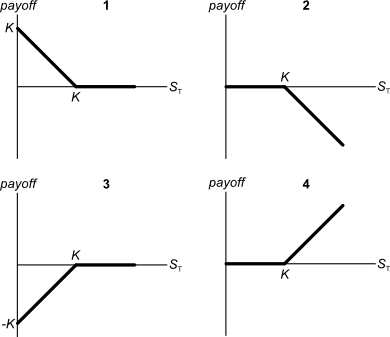# Fight Finance

#### CoursesTagsRandomAllRecentScores

The following equation is the Dividend Discount Model, also known as the 'Gordon Growth Model' or the 'Perpetuity with growth' equation.

$$p_{0} = \frac{c_1}{r_{\text{eff}} - g_{\text{eff}}}$$

What is the discount rate '$r_\text{eff}$' in this equation?

A European bond paying annual coupons of 6% offers a yield of 10% pa.

Convert the yield into an effective monthly rate, an effective annual rate and an effective daily rate. Assume that there are 365 days in a year.

All answers are given in the same order:

$$r_\text{eff, monthly} , r_\text{eff, yearly} , r_\text{eff, daily}$$

A three year project's NPV is negative. The cash flows of the project include a negative cash flow at the very start and positive cash flows over its short life. The required return of the project is 10% pa. Select the most correct statement.

A wholesale building supplies business offers credit to its customers. Customers are given 60 days to pay for their goods, but if they pay within 7 days they will get a 2% discount.

What is the effective interest rate implicit in the discount being offered?

Assume 365 days in a year and that all customers pay on either the 7th day or the 60th day. All rates given below are effective annual rates.

Below are 4 option graphs. Note that the y-axis is payoff at maturity (T). What options do they depict? List them in the order that they are numbered.A 30-day Bank Accepted Bill has a face value of $1,000,000. The interest rate is 8% pa and there are 365 days in the year. What is its price now? The boss of WorkingForTheManCorp has a wicked (and unethical) idea. He plans to pay his poor workers one week late so that he can get more interest on his cash in the bank. Every week he is supposed to pay his 1,000 employees$1,000 each. So \$1 million is paid to employees every week.

The boss was just about to pay his employees today, until he thought of this idea so he will actually pay them one week (7 days) later for the work they did last week and every week in the future, forever.

Bank interest rates are 10% pa, given as a real effective annual rate. So $r_\text{eff annual, real} = 0.1$ and the real effective weekly rate is therefore $r_\text{eff weekly, real} = (1+0.1)^{1/52}-1 = 0.001834569$

All rates and cash flows are real, the inflation rate is 3% pa and there are 52 weeks per year. The boss will always pay wages one week late. The business will operate forever with constant real wages and the same number of employees.

What is the net present value (NPV) of the boss's decision to pay later?

A stock has a beta of 1.5. The market's expected total return is 10% pa and the risk free rate is 5% pa, both given as effective annual rates.

Over the last year, bad economic news was released showing a higher chance of recession. Over this time the share market fell by 1%. So $r_{m} = (P_{0} - P_{-1})/P_{-1} = -0.01$, where the current time is zero and one year ago is time -1. The risk free rate was unchanged.

What do you think was the stock's historical return over the last year, given as an effective annual rate?

Alice, Bob, Chris and Delta are traders in the futures market. The following trades occur over a single day in a newly-opened equity index future that matures in one year which the exchange just made available.

1. Alice buys a future from Bob.

2. Chris buys a future from Delta.

3. Bob buys a future from Chris.

These were the only trades made in this equity index future. What was the trading volume and what is the open interest?

If a stock's expected future prices are log-normally distributed, what will be bigger, the stock's or future price? Or would you expect them to be ?